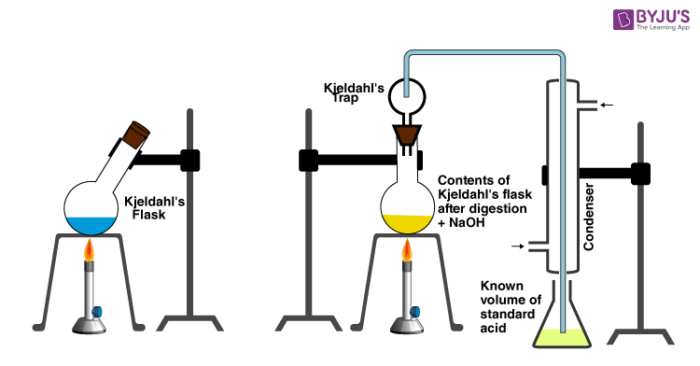# Kjeldahl Method

## What is Kjeldahl Method?

The Kjeldahl method first came into existence in 1883 and was developed by a Danish chemist named Johan Kjeldahl. This method was specifically developed for determining the nitrogen contents in organic and inorganic substances.

In today’s world, Kjeldahl nitrogen determinations are used on several samples like wastewater, soil, fertilizers, meat, feed, grain, and many other substances. The method is also used for estimating the protein content in foods.

## Equipment and Apparatus

The Kjeldahl method was first conducted using stone fume hoods and gas mantles. A few years down the line, macro-Kjeldahl digestion, as well as distillation instrument, was developed and used. The setup consisted of Kjeldahl flasks also. There is a miniature version of the setup known as micro-Kjeldahl equipment which consists of smaller sized units.In recent years, the equipment has further gone some significant changes and aluminium or ceramic heating blocks are used. This setup can even accept several straight digestion tubes at once. In addition, “Block digesters” are used alongside benchtop distillation units with steam generators in-order to decrease the distillation time. The equipment is mostly made of corrosion-resistant materials.

## Recommended Videos

#### Kjeldahl’s method and its Limitations#### Detection of Nitrogen & Sulphur## The Process of Kjeldahl Method

Since the development of Kjeldahl method about 100 years ago the technique and apparatus have been modified and made better. However, even with the new changes the basic principles introduced by Johan Kjeldahl are still relevant. The main objective or purpose of this procedure is the oxidation of organic compounds using concentrated sulphuric acid.

Overall, the Kjeldahl method is divided into three main steps. The method has to be carried out in proper sequence. The steps include digestion, distillation, and titration.

1. Digestion: In this method, a certain substance or sample is heated in the presence of sulphuric acid. The acid breaks down the organic substance via oxidation and reduced nitrogen in the form of ammonium sulphate is liberated. Potassium sulphate is usually added to increase the boiling point of the medium. Catalysts like mercury, selenium, copper, or ions of mercury or copper are also used in the digestion process. The sample is fully decomposed when we obtain a clear and colourless solution.

$$\begin{array}{l}Organic \, compound + H_{2} SO_{4} \rightarrow[digest]{Cu^{2+}} (NH_{4})_{2}SO_{4}\end{array}$$

2. Distillation: The distillation of the solution now takes place and a small quantity of sodium hydroxide is added to convert the ammonium salt to ammonia. The distilled vapours are then trapped in a special trapping solution of HCl (hydrochloric acid) and water.

$$\begin{array}{l}(NH_{4})_{2}SO_{4} + 2NaOH\overset{\Delta }{\rightarrow}Na_{2}SO_{4} + 2H_{2}O + 2NH_{3}\end{array}$$

3. Titration: The amount of ammonia or the amount of nitrogen present in the sample is then determined by back titration. As the ammonia dissolves in the acid trapping solution some HCl is neutralized. The acid that is left behind can be back titrated with a standard solution of a base such as NaOH or other bases.

$$\begin{array}{l}B(OH)_{2} + H_{2}O + Na_{2}CO_{3} \rightarrow NaHCO_{3} + CO_{2} + H_{2}O\end{array}$$
$$\begin{array}{l}NH_{3} + HCl \rightarrow NH_{4}Cl\end{array}$$

## Formula For Calculation

The percentage of nitrogen can be determined using the given formula:

$$\begin{array}{l}Percentage \: of \: nitrogen \: in \: the \: sample = \frac{1.4V\ast N}{W}\end{array}$$

Where,

• V = acid used in titration (ml)
• N = normality of standard acid
• W = weight of sample (g)

## Limitations of Kjeldahl Method

While the Kjeldahl method of nitrogen analysis has become the worldwide standard, this method is not suitable for compounds containing nitrogen in azo and nitro groups or in rings (quinoline, pyridine, etc.). In these cases, the nitrogen cannot be converted to ammonium sulphate by following the Kjeldahl method.

## Frequently Asked Questions – FAQs

### What is the principle of Kjeldahl method?

The Kjeldahl method was developed by a brewer called Johann Kjeldahl in 1883. The protocol is built on the principle that strong acid helps in the digestion of food so that it releases nitrogen which can be determined by a suitable titration technique.

### Which method is used to determination of nitrogen?

The Kjeldahl method is used to determine the nitrogen content in organic and inorganic samples. For longer than 100 years the Kjeldahl method has been used for the determination of nitrogen in a wide range of samples.

### Which indicator is used in Kjeldahl method?

One of the most used indicator solutions is the Tashiro indicator, added to the boric acid solution. The endpoint corresponds to a grey / pink colour. The acid titrant solution is added to the boric acid containing distilled ammonia manually by the analyst, using a glass burette.

### Why boric acid is used in Kjeldahl method?

The boric acid captures the ammonia gas, forming an ammonium-borate complex. As the ammonia collects, the colour of the receiving solutions changes. The boric acid method has the advantages that only one standard solution is necessary for the determination and that the solution has a long shelf life.

### What are the limitations of Kjeldahl method?

This method measures only nitrogen bound to organic components (proteins, amino acids, nucleic acids) and ammonium in the sample. This method is not suitable for compounds containing nitrogen in azo and nitro groups or in rings (quinoline, pyridine, nitrate, nitrite, etc).

Test your Knowledge on Kjeldahl method!